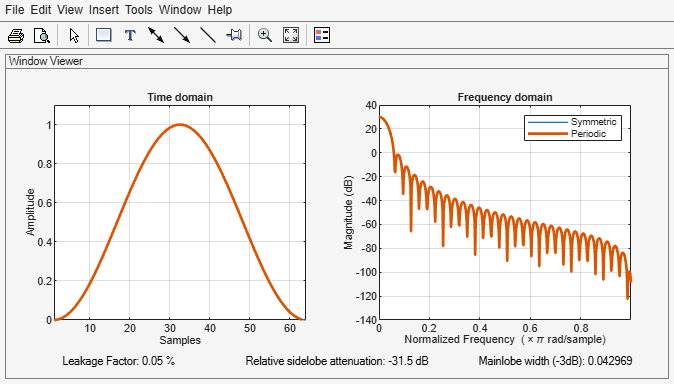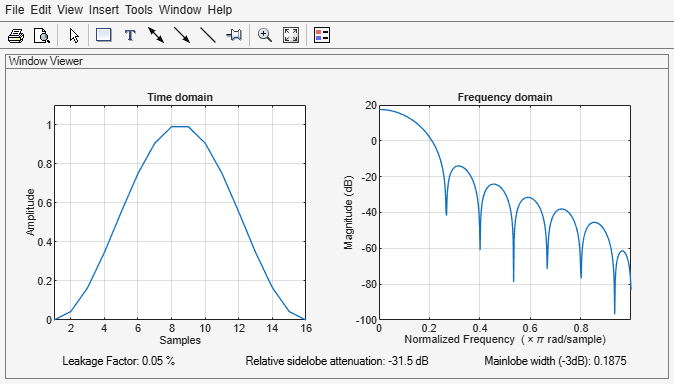Main Content

# sigwin.hann class

Package: sigwin

Construct Hann (Hanning) window object

## Description

Note

The use of `sigwin.hann` is not recommended. Use `hann` instead.

`sigwin.hann` creates a handle to a Hann window object for use in spectral analysis and FIR filtering by the window method. Object methods enable workspace import and ASCII file export of the window values.

The symmetric Hann window of length `N` is defined as:

`$w\left(n\right)=\frac{1}{2}\left(1-\mathrm{cos}\frac{2\pi n}{N-1}\right),\text{ }0\le n\le M-1$`

where M is N/2 for N even and (N + 1)/2 for N odd.

The second half of the symmetric Hann window $M\le n\le N-1$ is obtained by flipping the first half around the midpoint. The symmetric option is the preferred method when using a Hann window in FIR filter design.

The periodic Hann window is constructed by extending the desired window length by one sample, constructing a symmetric window, and removing the last sample. The periodic version is the preferred method when using a Hann window in spectral analysis because the discrete Fourier transform assumes periodic extension of the input vector.

## Construction

`H = sigwin.hann` returns a symmetric Hann window object `H` of length 64.

`H = sigwin.hann(Length)` returns a symmetric Hann window object with length `Length`. `Length` requires a positive integer. Entering a positive noninteger value for `Length` rounds the length to the nearest integer. Entering a 1 for `Length` results in a window with a single value of 1.

`H = sigwin.hann(Length,SamplingFlag)` returns a Hann window object with sampling `Sampling_Flag`. The `SamplingFlag` can be either `'symmetric'` or `'periodic'`.

## Properties

 `Length` Hann window length. Must be a positive integer. Entering a positive noninteger value for `Length` rounds the length to the nearest integer. Entering a 1 for `Length` results in a window with a single value of 1. `SamplingFlag` `'symmetric'` is the default and forces exact symmetry between the first and second halves of the Hann window. A symmetric window is preferred in FIR filter design by the window method. `'periodic'` designs a symmetric Hann window of length `Length+1` and truncates the window to length `Length`. This design is preferred in spectral analysis where the window is treated as one period of a `Length`-point periodic sequence.

## Methods

 generate Generates Hann window info Display information about Hann window object winwrite Save Hann window object values in ASCII file

## Copy Semantics

Handle. To learn how copy semantics affect your use of the class, see Copying Objects in the MATLAB® Programming Fundamentals documentation.

## Examples

collapse all

Generate two Hann windows:

• The first window has N = 64 and is symmetric.

• The second window has N = 63 and is periodic.

Display the two windows.

```Hs = sigwin.hann(64,'symmetric'); Hp = sigwin.hann(63,'periodic')```
```Hp = Name: 'Hann' SamplingFlag: 'periodic' Length: 63 ```
```wvt = wvtool(Hs,Hp); legend(wvt.CurrentAxes,'Symmetric','Periodic')```Generate a symmetric Hann window with N = 16. Return the window values as a column vector. Show information about the window object. Display the window.

```H = sigwin.hann(16); win = generate(H)```
```win = 16×1 0 0.0432 0.1654 0.3455 0.5523 0.7500 0.9045 0.9891 0.9891 0.9045 ⋮ ```
`wininfo = info(H)`
```wininfo = 4x26 char array 'Hann Window ' '----------- ' 'Length : 16 ' 'Sampling Flag : symmetric' ```
`wvtool(H)`## References

Oppenheim, Alan V., and Ronald W. Schafer. Discrete-Time Signal Processing. Upper Saddle River, NJ: Prentice Hall, 1989.

## Support

#### Deep Learning for Signal Processing with MATLAB

Download white paper